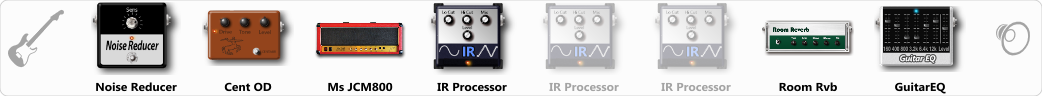# EMG 81 on the bridge

Discussion in 'ToneLib-GFX presets' started by NickyFingers, Jan 2, 2020.

1. EMG 81 on the bridge

Preset name: Boosted JCM800 2x12's

Effects chain:Effect: "Noise Reducer" (Dynamics / Filter), active - "yes"
"Sens" = 37
"Mode" = Soft

Effect: "Cent OD" (Overdrive / Distortion), active - "yes"
"Drive" = 52
"Tone" = 70
"Level" = 74

Effect: "Ms JCM800" (Amp simulators), active - "yes"
"Gain" = 100
"Bass" = 82
"Middle" = 23
"Treble" = 39
"Presence" = 65
"Master" = 78
"Output" = 45
"Level (dB)" = 0

Effect: "IR Processor" (Dynamics / Filter), active - "yes"
"IR" = V30 212 O Hi-Gn 121+57 Celestion
"Low Cut (Hz)" = 0
"Hi Cut (kHz)" = 20.0
"Mix" = 100
"Level (dB)" = 0

Effect: "IR Processor" (Dynamics / Filter), active - "no"
"IR" = V30 212 O MD421 Balanced Celestion
"Low Cut (Hz)" = 0
"Hi Cut (kHz)" = 20.0
"Mix" = 100
"Level (dB)" = 0

Effect: "IR Processor" (Dynamics / Filter), active - "no"
"IR" = V30 212 O MD421 Bright Celestion
"Low Cut (Hz)" = 80
"Hi Cut (kHz)" = 20.0
"Mix" = 100
"Level (dB)" = 0

Effect: "Room Rvb" (Reverb), active - "yes"
"Time" = 4.5
"PreDelay" = 0
"LoDamp" = 46
"HiDamp" = 32
"Mix" = 21

Effect: "GuitarEQ" (Dynamics / Filter), active - "yes"
"160 Hz" = -1
"400 Hz" = -4
"800 Hz" = -3
"1.6 kHz" = 0
"3.2 kHz" = 0
"6.4 kHz" = 0
"12 kHz" = 0
"Level (dB)" = 0

Note: You will need to download and install the ToneLib-GFX software to use the preset.

File size:
63.7 KB
Views:
4,192